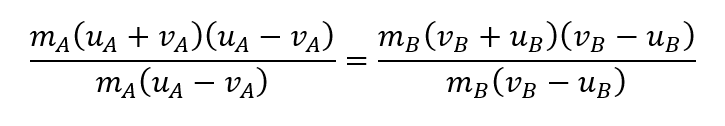cum
top of page
Search

# CAPE Physics: Motion Part 2 (Newton's Laws, Linear Momentum and Impulse)

Updated: Nov 25, 2021

This post will cover parts 8-16 of Unit 1 Module 1 Section 3 of the syllabus.

Where we left off in part one was with projectile motion, and a few equations that are used constantly in our calculations. Today, we'll take a look at linear momentum, Newton's Laws of motion and the concept of the impulse of a force.

Newton's Laws of Motion

First Law: Every object in uniform motion in a straight line or at rest will continue in that motion or in its state of rest unless acted upon by a net external unbalanced force. This tendency of an object to 'keep doing what it's doing' is called inertia. Mass is considered a measure of the inertia of an object.

Second Law: The applied resultant force on a mass is directly proportional to the rate of change of momentum of the mass and occurs in the direction of the change in momentum.

Expressing this law mathematically (note that ∆ means change in):But Quelpr-san! (v-u)/t is the equation for acceleration!You probably recognize this equation, and are wondering what that k is doing there. The S.I. unit for force is the newton (N), defined such that 1 N of net force gives a mass of 1 kg an acceleration of 1 ms^−2. This requires that k = 1. So,Third Law: If one object exerts force on another object, that object exerts an equal and oppositely directed force on the first. Or, every action has an equal and opposite reaction.

Linear Momentum

The linear momentum of an object is defined as the product of its mass and its velocity. The equation of linear momentum is as follows:From this equation, it's easy to see why larger (higher mass) objects have larger momentum. Since momentum and mass are directly proportional, an increase in one will result in an increase of the other. An object moving very fast will also have a large momentum, but this of course depends on mass (think of photons, which move at the speed of light, but have a theoretical mass of 0, or 10^-54 kg as a non-zero value in experiments).

Linear momentum is also a vector quantity since velocity is a vector quantity.

The Principle of Conservation of Linear Momentum

This principle states that the total linear momentum within a system (as long as no external forces act on it) remains constant, i.e. total momentum before = total momentum after. This can be shown in the following equation, where A and B represent arbitrary objects which collide with initial velocities of u and end with final velocities v.Which we can rearrange and factorize:(That last one's a surprise tool that will help us later)

Note- this equation holds true for all motion- 1, 2 and 3 dimensional motion- as long as the prerequisite of a closed system is met.

Elastic and Inelastic Collisions

These two forms of collisions vary based on what happens to the objects colliding after the collision. In an elastic collision, all kinetic energy is conserved. In an inelastic collision, kinetic energy is not conserved.

So, in equation form, an elastic collision will be represented like this:Elastic Collisions will usually have objects bouncing off of each other. Their kinetic energy is converted to potential energy then back to kinetic energy with 100% efficiency. There is no permanent deformation or 'sticking together' between the objects, nor is any other form of non-mechanical energy produced.

Inelastic Collisions will have the objects sticking to one another or experiencing permanent deformation. Work is done in causing damage or some other non-mechanical form of energy is liberated from the collision, such as heat or light.

So, for an elastic collision, the kinetic energy before and after is represented with the equation we used above:But lets take a deeper dive. Rearranging, we get:Since everything is multiplied by a half, we can take all those halves out of the equation (by dividing by a half) and get a simpler looking equation:Factorizing:From algebra, you should know that the difference of two squares can be factorized like this:If you remember our equation earlier from rearranging the equation for conservation of linear momentum (on the right), we can divide our current equation to get a pretty cool relationship unique to elastic collisions:Thus, in an elastic collision in a straight line, the velocities before and after collision are independent of the masses of the objects.

Impulse

If you remember from when we deriving F=ma, we got here:This also means that:This is known as impulse (J), or the duration of a force.The area under a force versus time graph (F vs t) is the impulse of a force on an object.